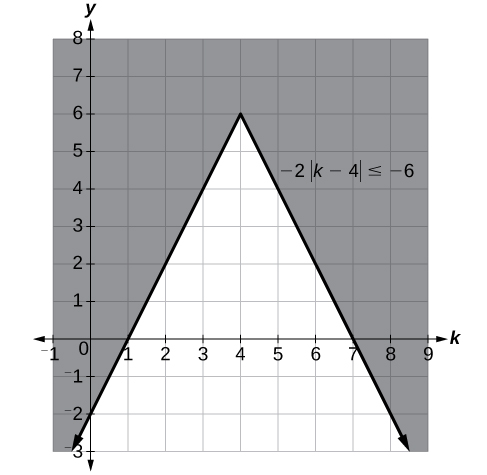# 2.7 Linear inequalities and absolute value inequalities  (Page 4/11)

 Page 4 / 11
$\begin{array}{c}-200\le x-600\le 200\\ -200+600\le x-600+600\le 200+600\\ 400\le x\le 800\end{array}$

This means our returns would be between $400 and$800.

To solve absolute value inequalities, just as with absolute value equations, we write two inequalities and then solve them independently.

## Absolute value inequalities

For an algebraic expression X, and $\text{\hspace{0.17em}}k>0,$ an absolute value inequality is an inequality of the form

These statements also apply to $\text{\hspace{0.17em}}|X|\le k\text{\hspace{0.17em}}$ and $\text{\hspace{0.17em}}|X|\ge k.$

## Determining a number within a prescribed distance

Describe all values $\text{\hspace{0.17em}}x\text{\hspace{0.17em}}$ within a distance of 4 from the number 5.

We want the distance between $\text{\hspace{0.17em}}x\text{\hspace{0.17em}}$ and 5 to be less than or equal to 4. We can draw a number line, such as in [link] , to represent the condition to be satisfied.

The distance from $\text{\hspace{0.17em}}x\text{\hspace{0.17em}}$ to 5 can be represented using an absolute value symbol, $\text{\hspace{0.17em}}|x-5|.\text{\hspace{0.17em}}$ Write the values of $\text{\hspace{0.17em}}x\text{\hspace{0.17em}}$ that satisfy the condition as an absolute value inequality.

$|x-5|\le 4$

We need to write two inequalities as there are always two solutions to an absolute value equation.

$\begin{array}{lll}x-5\le 4\hfill & \phantom{\rule{2em}{0ex}}\text{and}\phantom{\rule{2em}{0ex}}\hfill & x-5\ge -4\hfill \\ \phantom{\rule{1.8em}{0ex}}x\le 9\hfill & \hfill & \phantom{\rule{1.8em}{0ex}}x\ge 1\hfill \end{array}$

If the solution set is $\text{\hspace{0.17em}}x\le 9\text{\hspace{0.17em}}$ and $\text{\hspace{0.17em}}x\ge 1,$ then the solution set is an interval including all real numbers between and including 1 and 9.

So $\text{\hspace{0.17em}}|x-5|\le 4\text{\hspace{0.17em}}$ is equivalent to $\text{\hspace{0.17em}}\left[1,9\right]\text{\hspace{0.17em}}$ in interval notation.

Describe all x- values within a distance of 3 from the number 2.

$|x-2|\le 3$

## Solving an absolute value inequality

Solve $|x-1|\le 3$ .

$\begin{array}{l}|x-1|\le 3\hfill \\ \hfill \\ -3\le x-1\le 3\hfill \\ \hfill \\ -2\le x\le 4\hfill \\ \hfill \\ \left[-2,4\right]\hfill \end{array}$

## Using a graphical approach to solve absolute value inequalities

Given the equation $y=-\frac{1}{2}|4x-5|+3,$ determine the x -values for which the y -values are negative.

We are trying to determine where $\text{\hspace{0.17em}}y<0,$ which is when $\text{\hspace{0.17em}}-\frac{1}{2}|4x-5|+3<0.\text{\hspace{0.17em}}$ We begin by isolating the absolute value.

Next, we solve for the equality $|4x-5|=6.$

$\begin{array}{lll}4x-5=6\hfill & \hfill & 4x-5=-6\hfill \\ \phantom{\rule{1.9em}{0ex}}4x=11\hfill & \phantom{\rule{2em}{0ex}}\text{or}\phantom{\rule{2em}{0ex}}\hfill & \phantom{\rule{1.9em}{0ex}}4x=-1\hfill \\ \phantom{\rule{2em}{0ex}}x=\frac{11}{4}\hfill & \hfill & \phantom{\rule{2em}{0ex}}x=-\frac{1}{4}\hfill \end{array}$

Now, we can examine the graph to observe where the y- values are negative. We observe where the branches are below the x- axis. Notice that it is not important exactly what the graph looks like, as long as we know that it crosses the horizontal axis at $\text{\hspace{0.17em}}x=-\frac{1}{4}\text{\hspace{0.17em}}$ and $\text{\hspace{0.17em}}x=\frac{11}{4},$ and that the graph opens downward. See [link] .

Solve $\text{\hspace{0.17em}}-2|k-4|\le -6.$

$k\le 1\text{\hspace{0.17em}}$ or $\text{\hspace{0.17em}}k\ge 7;$ in interval notation, this would be $\text{\hspace{0.17em}}\left(-\infty ,1\right]\cup \left[7,\infty \right).$Access these online resources for additional instruction and practice with linear inequalities and absolute value inequalities.

## Key concepts

• Interval notation is a method to indicate the solution set to an inequality. Highly applicable in calculus, it is a system of parentheses and brackets that indicate what numbers are included in a set and whether the endpoints are included as well. See [link] and [link] .
• Solving inequalities is similar to solving equations. The same algebraic rules apply, except for one: multiplying or dividing by a negative number reverses the inequality. See [link] , [link] , [link] , and [link] .
• Compound inequalities often have three parts and can be rewritten as two independent inequalities. Solutions are given by boundary values, which are indicated as a beginning boundary or an ending boundary in the solutions to the two inequalities. See [link] and [link] .
• Absolute value inequalities will produce two solution sets due to the nature of absolute value. We solve by writing two equations: one equal to a positive value and one equal to a negative value. See [link] and [link] .
• Absolute value inequalities can also be solved by graphing. At least we can check the algebraic solutions by graphing, as we cannot depend on a visual for a precise solution. See [link] .

what is the function of sine with respect of cosine , graphically
tangent bruh
Steve
cosx.cos2x.cos4x.cos8x
sinx sin2x is linearly dependent
what is a reciprocal
The reciprocal of a number is 1 divided by a number. eg the reciprocal of 10 is 1/10 which is 0.1
Shemmy
Reciprocal is a pair of numbers that, when multiplied together, equal to 1. Example; the reciprocal of 3 is ⅓, because 3 multiplied by ⅓ is equal to 1
Jeza
each term in a sequence below is five times the previous term what is the eighth term in the sequence
I don't understand how radicals works pls
How look for the general solution of a trig function
stock therom F=(x2+y2) i-2xy J jaha x=a y=o y=b
sinx sin2x is linearly dependent
cr
root under 3-root under 2 by 5 y square
The sum of the first n terms of a certain series is 2^n-1, Show that , this series is Geometric and Find the formula of the n^th
cosA\1+sinA=secA-tanA
Wrong question
why two x + seven is equal to nineteen.
The numbers cannot be combined with the x
Othman
2x + 7 =19
humberto
2x +7=19. 2x=19 - 7 2x=12 x=6
Yvonne
because x is 6
SAIDI
what is the best practice that will address the issue on this topic? anyone who can help me. i'm working on my action research.
simplify each radical by removing as many factors as possible (a) √75
how is infinity bidder from undefined?ByByBy OpenStaxBy Brooke DelaneyBy Brooke DelaneyBy Richley CrapoBy OpenStaxBy Nick SwainBy Jonathan LongBy Lakeima RobertsBy CB BiernBy Tony Pizur